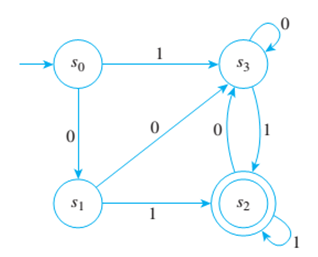Chapter 12.2, Problem 10ES### Discrete Mathematics With Applicat...

5th Edition
EPP + 1 other
ISBN: 9781337694193

#### Solutions

Chapter
Section### Discrete Mathematics With Applicat...

5th Edition
EPP + 1 other
ISBN: 9781337694193
Textbook Problem
1 views

# A finite-state automaton A given by the transition diagram below, has next-stale function N and eventual-state function N*.a. Find N ( s 1 ,   1 ) and N ( s 0 ,   1 ) . b. Find N ( s 2 ,   0 ) and N ( s 1 ,   0 ) . c. Find N * ( s 0 ,   10011 ) and N * ( s 1 ,   01001 ) .d. Find N * ( s 2 ,   11010 ) and N * ( s 0 ,   01000 ) .

To determine

(a)

Find N(s1,1) and N(s0,1)

Explanation

Given information:

Concept used:

Arrow in the given graph played vital role in answer selection.

Calculation:

N(s1,1)=s2 because s1 moves s2 through 1 arrow  N(

To determine

(b)

Find N(s2,0) and N(s1,0)

To determine

(c)

Find N*(s0,10011) and N*(s1,01001).

To determine

(d)

Find its accepting states.

Find N*(s2,11010) and N*(s0,01000).

### Still sussing out bartleby?

Check out a sample textbook solution.

See a sample solution

#### The Solution to Your Study Problems

Bartleby provides explanations to thousands of textbook problems written by our experts, many with advanced degrees!

Get Started